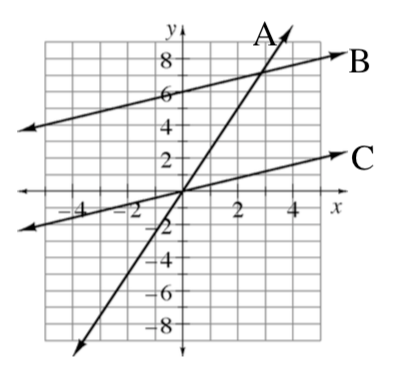Home > ACC7 > Chapter cc37 > Lesson cc37.2.2 > Problem7-49

7-49.

Compare the graphs of lines A, B, and C below. Homework Help ✎1. Which line has the greatest slope? Justify your answer.

Which line increases most sharply?

Line A

1. What is the $y$-value of line A when $x=2$?

Locate line A and $x=2$ on the graph.

What is the $y$-coordinate of the point on the graph?

1. Compare the slopes of lines B and C.

The lines are parallel. Parallel lines have the same change in $y$ for the same change in $x$.